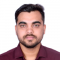## How to change the attributes of a networkx / matplotlib graph drawing?Published on 02-Feb-2022 11:56:11
To change the attributes of a netwrokx/matplotlib graph drawing, we can take the following steps −StepsSet the figure size and adjust the padding between and around the subplots.Initialize a graph with edges, name, or graph attributes.Add the graph's attributes. Add an edge between u and v.Get the edge attributes from ... Read More

## How to fill an area within a polygon in Python using matplotlib?Published on 02-Feb-2022 11:47:09
To fill an area within a polygon in Python using matplotlib, we can take the following steps −StepsSet the figure size and adjust the padding between and around the subplots.Create a figure and a set of subplots.Get an instance of a polygon.Get the generic collection of patches with iterable polygons.Add ... Read More

## How to get data labels on a Seaborn pointplot?Published on 02-Feb-2022 11:37:50
To get data labels on a Seaborn pointplot, we can take the following steps −StepsSet the figure size and adjust the padding between and around the subplots.Create a dataframe, df, of two-dimensional, size-mutable, potentially heterogeneous tabular data.Create a pointplot.Get the axes patches and label; annotate with respective labels.To display the ... Read More

## How to draw a precision-recall curve with interpolation in Python Matplotlib?Published on 02-Feb-2022 11:33:00
To draw a precision-recall curve with interpolation in Python, we can take the following steps −StepsSet the figure size and adjust the padding between and around the subplots.Create r, p and duplicate recall, i data points using numpy.Create a figure and a set of subplots.Plot the recall matrix in the ... Read More

## How to plot additional points on the top of a scatter plot in Matplotlib?Published on 02-Feb-2022 11:29:47
To plot additional points on the top of a scatter plot in matplotlib, we can take the following steps −StepsSet the figure size and adjust the padding between and around the subplots.Make a list of x and y data points.Create a scatter plot with x and y data points.Plot the ... Read More

## Transparent error bars without affecting the markers in MatplotlibPublished on 02-Feb-2022 11:26:04
To make transparent error bars without affecting markers in matplotlib, we can take the following steps −StepsSet the figure size and adjust the padding between and around the subplots.Make lists x, y and z for data.Initialize a variable error_bar_width=5Plot y versus x as lines and/or markers with attached errorbars.Set the ... Read More

## How to set legend marker size and alpha in Matplotlib?Published on 02-Feb-2022 11:21:30
To set legend marker size and alpha in matplotlib, we can take the following steps −StepsSet the figure size and adjust the padding between and around the subplots.Initialize a variable N to store the number of sample data.Plot the x and y data points with marker="*".Place a legend on the ... Read More

## Showing points coordinate in a plot in Python MatplotlibPublished on 02-Feb-2022 11:17:43
To show points coordinate in a plot in Python, we can take the following steps −StepsSet the figure size and adjust the padding between and around the subplots.Initilize a variable N and create x and y data points using numpy.Zip the x and y data points; iterate them and place ... Read More

## How to unset 'sharex' or 'sharey' from two axes in Matplotlib?Published on 02-Feb-2022 11:13:03
To inset sharex and sharey from two axes in matplotlib, we can use 'none', i.e., False or 'none'. Each subplot X- or Y-axis will be independent.StepsSet the figure size and adjust the padding between and around the subplots.Initialize two variables rows and cols.Create a figure and a set of subplots.Iterate ... Read More

## How to obtain 3D colored surface via Python?Published on 02-Feb-2022 10:55:15
To obtain 3D colored surface via Python, we can take the following steps −StepsSet the figure size and adjust the padding between and around the subplots.Create x and y data points using numpy.Get 3D data, i.e., z.Create a new figure or activate an existing figure.Get the 3D axes.Create a surface ... Read More